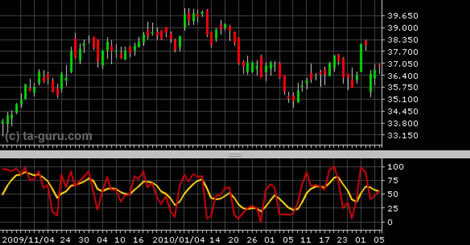Chart Pro online
User name (email):

 Recently used

 Forex

 Indices

# Stochastic K%D

Stochastic indicator is indicator in technical analysis created by George Lane. It belongs to oscillators and measures the relative position of the closing prices compared to the amplitude of price oscillations in a given period. The indicator is based on the assumption that as prices rise, the closing price tends towards the values that belong to the upper part of the area of price movements in the preceding period. When prices fall, the opposite is true.

Formulas for calculating the % K and % D lines are:

 %K =%D = SMA (%K, 3)

L (n) is the lowest low and H (n) is the highest high value in last n samples. For n is usually taken value of 14.

Lines %K and %D obtained using the above defined formula are called fast lines (%K fast, %D fast). %K slow is obtained by calculating SMA of %K fast, while formula for %D fast remains the same except it is applied on %K slow.Stochastic K%D (slow)

Interpretation

Stochastic K%D indicator belongs to the oscillators and its value is limited between 0 and 100. Values of %D line that are above 80 indicate that the security is overbought and the values below 20 that it is oversold. But often happens that when the value exceeds 80 prices continue to rise. Therefore, traders more often are using divergence as criteria for buying and selling signals.

If price of security falls to new low, but K%D fails to reach new low, downward trend is losing its power and we could expect trend reversal in the near future. Similar goes for upward trend: if K%D fails to reach new high when price hits new high, upward trend is losing its power.

Buying and selling signals can also be generated when lines %K and %D are intersected: when %K crosses above %D, buying signal is generated and when the %K crosses below %D, selling signal is generated. However, due to frequent intersections false signals could be generated.

Example